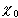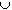#Interactive Real Analysis

Next | Previous | Glossary | Map

## 2.2. Uncountable Infinity

### Example 2.2.7: A Hierarchy of Infinity - Cardinal Numbers

When dealing with cardinal numbers, one can establish the following rules and definitions:
1. Definition of a cardinal number
2. Comparing cardinal numbers
3. The power of the continuum and the cardinality aleph null
4. Addition of two cardinal numbers

### 1. Definition of Cardinal Number

• Two sets A and B are called equivalent if there exists a bijection between A and B. The two sets are said to have the same cardinality, or power.
• The cardinality of a set A is denoted by card(A).
• The cardinal numbers of two sets are equal if the sets are equivalent.
Note that for finite sets the cardinality is just the number of elements in that set. That is, card({a, b, 2} ) = card({1, 2, 9}) = 3. However, the concept of cardinality also applies to infinite sets.

### 2. Comparing Cardinal Numbers

• A cardinal number c is less than or equal to another cardinal number d if there exist two sets A and B with card(A) = c and card(B) = d and card(A)card(B)
Note that according to this definition, we have that the cardinality of the natural numbers is strictly less than the cardinality of the real numbers. In fact, those cardinalities have a special name:

### 3. Special Cardinalities

The cardinality of the real numbers is called the cardinality (or power) of the continuum, and is denoted by
• c = card(R).
The cardinality of the natural number is called aleph null and is denoted by
•= card(N)

Let c and d be two cardinal numbers and take sets A and B with card(A) = c and card(B) = d. Define the sets
• A' = {(a, 0) : aA}
• B' = {(b, 1) : bB}
Then the sum of the two cardinal number c and d is defined as
• c + d = card(A'B')
Note that the reason for defining the sets A' and B' is to make sure that the resulting sets are disjoint. If the two sets A and B are disjoint from the outset, one could define the sum of the cardinal numbers as the cardinality of the union of the original sets.

### Example:

Let A = {1,2,3} and B = {1,2}. Then card(A) = 3 and card(B) = 2. However,
• card(AB) = 3
because AB = {1,2,3}. Using the above definition, we get:
• A' = { (1,0), (2,0), (3,0)}
• B' = { (1,1), (2,1) }
therefore
• A'B' = {(1,0), (2,0), (3,0), (1,1), (2,1)}
and therefore, as one would think:
• card(A'B') = 5 = card(A) + card(B)
Next | Previous | Glossary | Map